# The axle of an automobile is acted upon by the forces and couple shown. Knowing that the diameter of the solid axle is 32 mm. determine (n) the principal planes arid principal stresses at point H located on top of the axle. (b) the maximum .shearing stress at the same point.

Question-AnswerCategory: Strength of MaterialsThe axle of an automobile is acted upon by the forces and couple shown. Knowing that the diameter of the solid axle is 32 mm. determine (n) the principal planes arid principal stresses at point H located on top of the axle. (b) the maximum .shearing stress at the same point.

The axle of an automobile is acted upon by the forces and couple shown. Knowing that the diameter of the solid axle is 32 mm. determine (n) the principal planes arid principal stresses at point H located on top of the axle. (b) the maximum .shearing stress at the same point.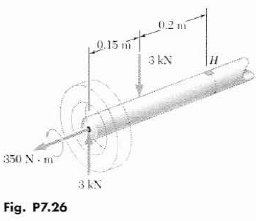Step No: 1

(a)
Draw the diagram representing the direction and magnitude of the forces acting on the solid axle: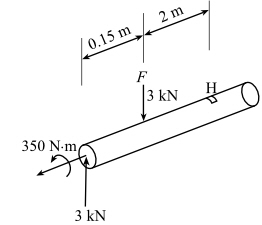Step No: 2

Calculate the radius c of the solid axle using the following equation: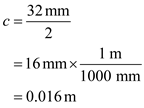Calculate the polar moment of inertia J of the solid axle using the following equation: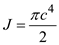Substitutefor c.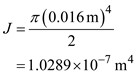Calculate the moment of inertia I of the solid axle using the following equation:Substitutefor c.Step No: 3

Calculate the shear stress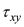acting on the point H using the following equation: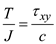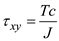Here the torque of the solid axle is T.
Substitutefor T,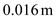for c, and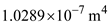for J.Calculate the bending moment M of the load F acting on the solid axle using the following equation:Substitutefor F.Step No: 4

Calculate the x component of the direct stressacting at point H due to the load F using the following equation: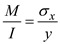Substitute c for y.Substitute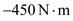for M,for c, and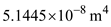for I.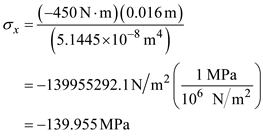Step No: 5

Draw the diagram representing the stresses acting at the point H:Step No: 6

As there is no direct stress acting in y direction, the stress in that direction will be zero i.e..
Calculate the angle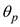of the principal planes at point H using the following equation: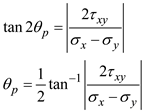Substitute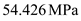forfor, and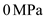for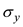.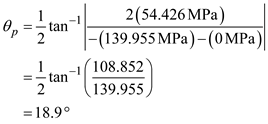Step No: 7

Therefore, the angle of the principal plane at point H is.

Step No: 8

As there are two principal planes which are perpendicular to each other, calculate the angle of the perpendicular principal plane using the following equation: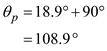Therefore, the angle of the perpendicular principal planes is.

Step No: 9

Calculate the maximum principal stressacting on the principal plane using the following equation:Substitutefor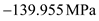for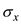, andfor.Therefore, the maximum principal stress acting on the principal plane is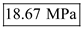.

Step No: 10

Calculate the minimum principal stress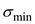acting on the principal plane using the following equation: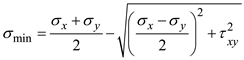Substituteforfor, andfor.Therefore, the minimum principal stress acting on the principal plane is.

Step No: 11

(b)
Calculate the maximum shearing stressacting on the point H using the following equation: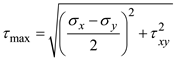Substituteforfor, andfor.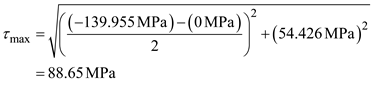Therefore, the maximum shearing stress acting on the point H is.